## miércoles, 9 de mayo de 2012

### Curves and surfaces. Generation and classificationhttp://teoremas-de-geometria.blogspot.com.es/2012_03_01_archive.html

curves and elements.

A moving point describes a curve, so that it can be defined as the locus of the different positions of a point it moves.
When the moving element is a line generates a surface, it can be defined as the locus of the different positions of a line.
A surface generally is also part of a body boundary, the term the surface, is something that is in contact with the outside, with the surrounding space. On the surface only two dimensions can be considered as it has no volume.

The curves can be flat or warped
If the point generating the curve moves in the plane creates a plane curve, if you do out of the plane curve warped. Warped curve is also called double-curved.
element is rectilinear segment connecting two points infinitely close of the curve.
Drying is the segment joining two distant points of the curve.
tangent is the limit of the secant of two points infinitely close . Is the prolongation of the rectilinear element.
Normal is perpendicular to the tangent line drawn at a point on the curve.
contingency is that angle defined by two tangents to the curve.
osculating circle is that which coincides with three points of the curve.
Center of curvature is the center of the osculating circle.
radius of curvature is the osculating circle.
total curvature angle contingency extreme tangent of the curve.
http://curvas-planas.blogspot.com/
http://curvas-conicas.blogspot.com/

Definition of surface: A surface is the locus of the different positions of a line or curve in space. Is the envelope surface which surrounds a body. The planes are directors or guidelines which establish the movement of the generatrices of the surface. May be limited or unlimited as containing a finite volume within a body or not. Tangential surface is one that has a common line as other surface, without cutting it or through it.
flat surface is one in which you can draw straight lines in any direction from it.
Surface casing is what generates various positions in another area called involute .

Ruled surfaces are generated by movement of a line and may be followed by a ruler so that it touches all the points on the surface through one of its generatrices.Ruled surface is one that can be followed with a rule on all sides touching all points.
cylindrical surface is one that generates a line when moving always parallel to a given direction.
The conical surface is generated by a straight line that moves always affecting the same fixed point and a guideline curve. (Guide line is that line by passing all the generators and the generators are the lines that determine the surface). The surface is regulated to be comprised of cylinders (3-cylinder intersection equal concurrent orthogonal axes). The cylinders are surfaces whose generatrices can be followed with a ruler touching all points.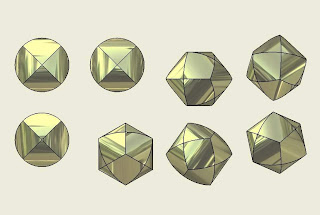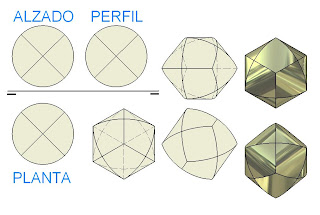Developable surfaces are those which can be extended onto a plane without deformation, such as cylindrical and conical surfaces. Two infinitely near generatrices intersect. If a plane tangent to the surface at a point as is throughout the generatrix passing through that point,
generally any of these properties are sufficient to be considered developable surface. radiated surfaces are generated by movement of a line supported by a proper or improper point and a line or curve. warped surfaces are those that can not develop and have their generating infinitely close. The warped surface which is being regulated (which means it can be done with straight lines) can not be developed (which means it can not be extended on a plane without distortion). A tangent thereto at a point contains its generatrix but is not tangent to the surface at other points of the generatrix. warped surfaces are generated by moving a straight line so that two adjacent positions on a line intersect. In the figure a set of straight lines are supported by two others, called directives, generating a warped ruled surface. A warped curve is a line in which all points are not on the plane. Surface warped and warped ruled surface are synonymous, are used interchangeably.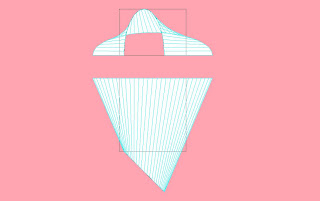Splayed MarseilleClassification of warped surfaces:
the generators should always be based on three guidelines: 1 - based on three guidelines without ever losing contact with them. In this case we have the elliptical hyperboloid of revolution, built on three lines straight. warped curves constructed with two lines and a curve.

Warped curves constructed with three curved lines.Warped curves constructed with a straight line and two curves, for example the cow horn or step sideways . 2 - based on two guidelines and are always parallel to a plane director.Supported on two straight lines have the hyperbolic paraboloid. Supported a straight line and a curve we have the conoid and straight helix. Supported by two curved lines have the cylindroid. 3 - based on two guidelines and form the generating always the same angle with a plane.Supported by two straight lines have the hyperboloid concoideo Leaning on a straight line and a curve we have the oblique helix.Supported by two curved lines have the oblique helix.All warped surfaces are always regulated, meaning that can be generated by a straight line. If a point of a ruled surface can only draw a straight line and no more is said that the ruled surface is simple, also called single or simple formal setting. If you can draw two will be dual adjustment. Double Regulating surfaces are the hyperboloid elliptic and hyperbolic paraboloid.

The hyperbolic paraboloidThe paraboloid is generated by a line that is based on two guidelines and always remains parallel to a plane called director. Another set of guidelines and considered as generating a plane parallel to the guidelines defined as a new master plan.
Two infinitely near generatrices intersect while the system is cut differently.
The surface is second order because if it is cut by a straight cutting at most two points.
The tangent plane at a point to it is defined by two generatrices, one of each system, and both pass through the plane.
Because each system contains a generator in the infinite-infinite line all director-level cutting plane has two points at infinity in common with the surface. The flat sections of the surface are generally hyperbolas and parabolas in particular cases.
planes parallel to the line common parabolic sections produce master plans while all other sections are hyperbolic.

The conoid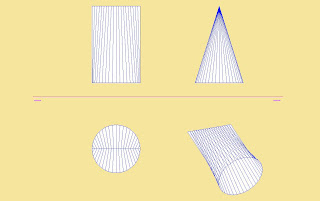The conoid is a ruled surface with a flat head bent and two directives, one straight and one curved. If the directrix curve is a circle has a conical circular, an ellipse if we have the elliptic conoid, etc..
If the line directrix is parallel to the plane of the directrix curve and perpendicular to the surface generated Director conoid is called Straight, If it is not, is called oblique. conoid Different sections of the conoid, from the triangle CC, AA to the ellipse, the shape aerodynamic drop of water in BB, to approximate curves in form of links of different ellipses: DD and EE. Hyperboloid of revolution of a branch. Also called hyperboloid and is a special case of elliptical hyperboloid. All sections that cut the surface perpendicular to the axis are circles. The hyperboloid can be generated by a straight line always moves in contact with three guidelines that cross, also by a line revolving around the axis so that it intersects. Also be generated by a line that moves incident on three circles whose centers are on the axis of revolution. Can also be generated by a hyperbola revolves around the leader.As Double Regulating the hyperboloid can be constructed by straight cross bar. It is applied in towers, masts, tissues, hyperbolic gear for two intersecting axes. The road surfaces are hyperboloids trunks. So are the gear teeth hyperbolic spiral action to smooth gear drive system. If we consider two lines that intersect one of which is the axis of rotation when rotated, it generates a hyperboloid of one sheet. The straight infinitely close to this surface and intersecting any symmetrical with respect to a meridian plane of the surface of revolution is a generatrix of the other system of lines. The hyperboloid is a surface whose sections are always conical surface when the generatrix rotates any appears twice parallel to a meridian plane so that every meridian section is a hyperbola. It follows that the surface can be generated by rotation of a hyperbola around its axis. The hyperboloid surface is a second order and for each one of its two lines pass each set defining a plane tangent to points. This sectioned plane to the surface in two straight lines. The surface can not develop because warped. To calculate the intersection of a plane with a warped surface are the intersection points of the generatrix with the cutting plane and then joined together. The intersection of any surface is warped with another obtained by calculating the intersections of the generators of the two. The twisted ruled surfaces are a very widespread in the construction of decks, roofs, plumbing adjustments, gears, cooling towers of nuclear power plants to adjust hyperbolic gear wheels whose axes intersect , etc. The hyperbolic paraboloid is a ruled surface warped, can be constructed with straight lines and at the same time is of double curvature.Two lines very close to the cross and hyperbolic paraboloid is a surface that can not be developed. The hyperbolic paraboloid is constructed of straight lines which are based on parables, these guidelines are the curves of the surface. The hyperbolic paraboloid is the shape of a saddle and has all its points hyperbolic, meaning that the environment of a point, two planes normal to the surface can be generated as a curve section concave and one convex. Generally all sections of this surface are hyperbolas, except those caused by incidents or sections of planes parallel to the intersection of the planes directors. http://superficies-regladas-alabeadas com /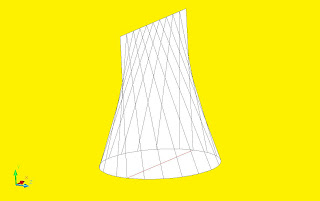A warped curve is one whose points are not on a flat surface which is bent to be regulated can not be developed, ie which can not be extended onto a plane.Another hyperbolic paraboloid , but with less curvature, is formed by straight lines ( generatrices) supported by two lines that intersect ab (guidelines).The parallel planes containing these lines determine one of the directors planes, the other is perpendicular to both. A director plane is the plane surface can always be maintained parallel to a generatrix thereof. Directors of the two planes is apparent that the surface has two distinct generatrices of which are cut, whereas the same system parallel to a principal plane intersect. Two sections of a different shape with hyperbolic paraboloids faces . If the cutting plane coincides with a shift in its master plan we have as a polygon section, if the cut is oblique usually have a shape formed by hyperbolas. The geometry of the universe in a hyperbolic paraboloid. The space in which we operate is a flat space, the parallel in the strict sense and not cut the angles of a triangle add up to 180 °, and so on. Two other geometries that correspond to all points in space are uniform and comply with the principle of isotropy, that the universe is equivalent in all directions. Apart from the geometry of flat space then there are two other possibilities, which is spherical or which is hyperbolic. In the first curvature is positive means that in the neighborhood of a point the two curves which pass through it are convex. In a spherical universe the shortest distance between two points is a geodesic, a circle whose center is the area. Thus lines are always parallel to the cut end, the angles of a triangle always add up to more than 180 ° and the circumference of a circle is less than the length of the circumference of the plane. As the area which it operates, which closes on itself, there is a finite space because the surface is too. In the case of hyperbolic paraboloid which finds its application in a hyperbolic universe parallels no longer cut, the triangles being the concave surface are less than 180 ° circumference and deposited on this universe shaped saddle is greater than the length of the circumference on the plane. Euclidean plane in space, if you increase the elements of infinity we obtain the projective plane, where we have that space contains a line at infinity. Hyperbolic space as the plane is infinite.These spaces generate different perspectives and distort the appearance of the cosmic microwave background. Those who are more undulating in the background are all the same size, regardless of the inflation process specific. The Universe being a plane, the larger waves could be the size of 1. If the universe is hyperbolic could reach half a degree according to the distortion of light rays. It seems that after several observations ripples acquire measures the size of 1 with what is coming down the open inflationary theory. If we represent the three models of space in three dimensions is necessary to make a point of view and shows the same a reference system. The outside view of the space surface serves to derive basic geometric rules while the internal space defined sizes references between objects at different distances. In flat space we have due to the perspective of angular size equal spheres is inversely proportional to distance, which leads us into the projective space of vanishing point, the point which has its counterpart in the infinite . The spherical space containing internal distances increase and then collapse into the opposite pole, is a system based in dodecahedra. negative curvature space being of sharp angles, there is a greater angular decrease in Euclidean space. Its network at each vertex contains five cubes. Surface composite is that generated or formed by the combination of others.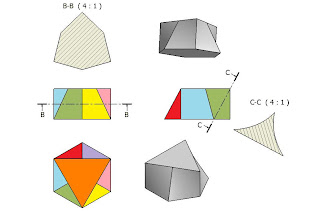Tangent to a surface.
The tangent to a surface at a point is the tangent at that point in any corner of the surface.
The tangent plane to the surface at a point is the locus of all tangents passing through that point.
a plane normal to a surface at a point when it is to the plane tangent to the surface at the same point.

Curved surfaces are those that are generated by the movement of a curve but which can not develop or warping.

Double-curved surfaces are generated by the movement of a curve. There are two types: the surfaces of revolution generated by the movement of a curve which revolves around an axis and the surfaces of progress or development that are generated by the movement of a curve which can be variable and along a path that is not circular. All surfaces of double curvature that are not of revolution, are evolving. The surface of revolution generated by a line or curve which rotates around a line called the axis of revolution, both being in the same plane.

Surfaces of revolution of double curvature: a cone is a surface of revolution because it engenders a line revolves around an axis of revolution but not double-curved because the spinning is a straight and a curved line.
The sphere yes that is a surface of revolution as a double curve is a curve that revolves around one of their diameters.
All conic curves generated by rotating the cone about an axis of revolution surface of double curvature, the ellipse generated by the ellipsoid , the hyperbola the hyperboloid, the circumference the sphere or torus, the parable of the paraboloid, and so on.
If we take irregular shapes have complex surfaces of revolution such as vases, lamp bases etc. double-curved surfaces are not developable, but can be approximated by developing cylindrical surfaces between generatrices.
All sections orthogonal to the axis of revolution are always circles called parallel, while sections passing through the axis of revolution are meridians.

Surfaces of evolution:As the name refers, are surfaces that curves or lines evolve differently. Surface evolution is that which evolves or transforms, being generated by the binding of different curves. In the figure a square becomes uniformly in a circle. For digital construction using the loft command. Its appearance is sometimes an impossible form of a figure with a perspective out of drawing-as appears to the drawing.
As an example of surfaces of evolution or development have automobile bodies, airframe, hull vessels and irregular surfaces are typically complex and smooth rounded contour and whose definition is not sufficient to define them. The way to define them is through major projections or major sections between parallel planes.It is analogous to what is done in the bounded system , represent a mountain or contour lines of level, this is an example of the method also has been doing for years for shipbuilding. Design ships: these surfaces contoured sections are generated by planes orthogonal to the projection of the set of horizontal and vertical planes, determining points of the projected surface and generating, for example, in a hull of a boat, the waterline in elevation and in the plant and the frames of vertical planes or sections in the profile. Each frame is shown in vertical projection is the intersection of the surface of the vessel with a vertical and a horizontal plane. Water lines indicated in a plan projection represent curves of intersection with horizontal planes. should be made ​​deep study of the fairing so that the final surface is smooth.To achieve the curves must have a very large radius of curvature and conical be used, which have to have the property of being smooth, it means that not noticeable transition from one to another. Surfaces generated by conics are fuseladas andaerodynamic .

We show that projective geometry to determine a conic five elements are needed between points and / or tangents. The tangents are useful to define the slope at each point of the conic. In this way the points of the outlines of the surfaces can be joined by a five conical elements and then bind with another conical.

The helix
is a double-curved line that is generated by a point which moves uniformly around an axis while moving always parallel thereto.
is the result of composing a rotation and translation , if the point always equidistant from the axis, the propeller is cylindrical while if the distance from the axis varies uniformly as it moves the propeller, is conical. Can also be considered as a propeller rotating circumference while moving in the axial direction. When the circumference gives a complete revolution is called the pitch of the propeller. You can rotate clockwise or counterclockwise.
The main application of this curve is the screw also used in cylindrical and conical springs, drills, rock drills spiral staircases, etc..
The propeller is the motion that describes point of a propeller of an airplane as it moves, it is also the guideline of helical surfaces.
To build it we use three data, the diameter, the pace and direction of rotation of the propeller. To draw enough to make circles at different heights and generating an elevation of a cylinder projected onto the raised floor. The intersection of the first line with the first circle is a point on the curve, the second line with the second circumference another point on the curve, and so on. The more circles and lines have the more accurate figure will curve drawn. As circumferences are equidistant angular divisions are equal to the generatrices, we have that the angle of inclination of the helix is constant. The development of the figure is a triangle, the base of the triangle is the rectification of the base circle of the cylinder, the height is the step length and the hypotenuse of the triangle is the true magnitude of a full rotation of the propeller. The angle between the hypotenuse and the base is always a constant slope. The slope is the tangent of the angle and to determine its inclination divide the passage between the length of the circumference, or whatever it is, the height of the triangle divided by the circumference length. If we draw the tangent to the curve, we must know who has the same slope as the propeller as coinciding with the development of it. Helicoide or propeller adapted to a bull or "donuts" called O-helix. The propeller can be adapted adopting any surface plus the proper name of the surface in which is accommodated. helizoidal surface is generated by a line revolving around an axis while moving in the direction thereof. If we take a helicoid and one of its points put a line with any position, displacement of the line by the helix generates a helizoide. The helix is the curve that generates a point when composing a turning movement plus another translation, is the movement describing a point of a propeller aircraft when moving. If the point describing the helix or helix joined at its axis of revolution with straight lines have a helical surface helizoide call, if these straight lines are perpendicular to the axis have a straight helicoid. The helix is a particular example of the handrails or edge of the steps of a spiral staircase. http://helicoides.blogspot.com/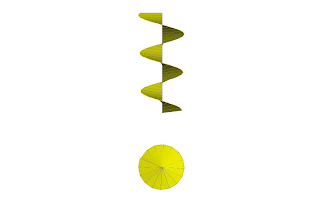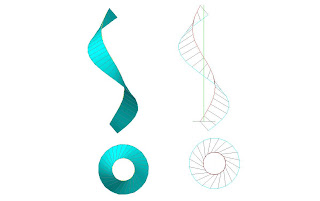The lines that pass through the helix can be oblique to the axis, which have an oblique helicoid. They can cut the shaft or can interbreed with it can produce twisted ruled surfaces. The helicoids are adaptable to any type of surface, in the first case (upper left) adjusted to an ellipse of revolution generated by an ellipsoid, which have an elliptical helix. In the second case is appropriate for a bull without a hole (a Donette in red) in the third case in blue that is adapted to have a cone of revolution. If a spiral ( http://curvas-planas.blogspot.com/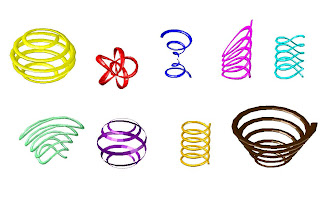) Is stretched uniformly orthogonal to one of its vertices away from the plane in which is housed, we obtain a conical helix.
In magenta have a spring made ​​by one edge in what we blue with a twist, etcetera. When Instead of moving a point to generate the helix, which moves is a sphere, we have an object called a coil . As the coil spring is a movement whose cross sections of the area that generates the surface are circles have also engenders when moving along a circumference of the helical trajectory of the helix being the points of the circumference centers and this orthogonal to the axis of displacement. A helical convolute is the surface generated by a straight line which is tangent to the helix. Need to define helical given guideline and a point of tangency. This surface may be placed between two concentric cylindrical surfaces in the plant to determine the two points of intersection of each line with the cylinders and obtain the contour of the two which are helicoids guidelines of the surface. The right helicoid is a ruled surface whose generating warped moves always in contact with two concentric helices. These propellers are guidelines and form an angle with their axes always equal. If the generator is orthogonal we have a right helicoid, if not we have an oblique. The helix has a generating straight shaft incident falls under the classification of the conoid, since all elements are parallel to the plane director. The oblique helicoid is one whose generatrix is always moved by the same angle. The helical surface has many applications, the square thread screw has a lateral surface which is a straight helicoid, the helical spring windings. The threads of screws, coil springs, springs, threads drills, spiral staircases, etc..Radiating surfaces: cones, cylinders, prisms and pyramids.

A cone with vertex at infinity is a cylinder , a pyramid with a vertex at infinity is a prism, a prism is a cylinder infinite faces are all special cases of the cylinders. The cylinder is a surface of revolution and regulated . Radiating surfaces are ruled (that means that if we take a generator of it we can follow the entire surface with the edge of a ruler) and developable (can be extended on a plane) may also be cylindrical or prismatic if the contact point the rule is at infinity. The cylinder is also a radiated surface means that if we take a generator of it we can follow the entire surface with the edge of a ruler and a point on the edge of this rule always passes through a point fixed surface, ie that the rule touches a point on the shaped surface and ideal invariably if at infinity, as the case of the cylinder or prism. A prism obliquely to the left and one right rectum. The displacement or extrusion of a polygon in a straight line or oblique prism generates a straight or oblique, respectively. The prism is another type radiated cylindrical surface because the lines connecting their bases are connected by parallel lines. The radiated cylindrical surface is a special case of the conic in which the vertex through which pass the rays is at infinity. Prismatic surface is generated by a straight line always moves parallel to another and a polygonal broken path is on a different plane to it. The cone is a cone-type radiated surface that is generated by revolving a straight about an axis, both in the same plane. The directrix line or curve which intersects all the generatrices and is in this case a circle. The guideline curve is a section of the cone. The pyramid is another type of surface radiated cone type because it has its vertex located at a point itself. The guideline is a regular polygon or irregular pyramid is straight and if its vertex affects the line perpendicular to the base, if not, as in this case, an oblique pyramid. Is a ruled surface, since a rule pass through its vertex can follow all the faces of the surface, and is also developable, meaning that their faces can be extended on a plane and to build paper.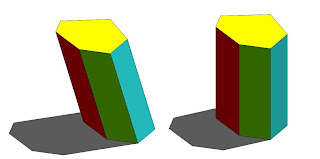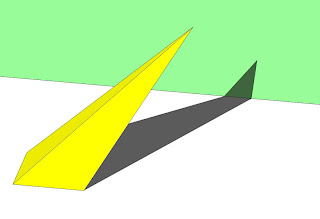surface of revolution is generated by rotating a line or curve around another line called the axis of rotation, both being coplanar.
If we cut the surface by a plane orthogonal to the axis parallel we call a circumference. Every surface of revolution having a radius parallel maximum and minimum, respectively called circles of Ecuador and throat.
Any plane that cuts the figure and the axis that affects the surface of revolution is called meridian. The meridian frontal plane is that which corresponds to the contour of the surface elevation.Meridians are curved lines symmetrical with respect to the axis of revolution, and the surface is always symmetrical with respect to any meridian plane passing through the axis.
Each point on the surface contains a parallel and a meridian, except where the surface intersects the axis in a point, in this case pass through the point all meridians.
entire surface of revolution is defined by the generatrix curve or line and its axis, both being one same plane.
Any plane tangent to the surface of revolution is defined by lines as people with two surface curves passing through it.
As a particular case we define the tangent meridian and parallel passing through a point.
The tangent plane at a point is perpendicular to the meridian plane passing through the point. All meridians and parallels intersect each other perpendicularly.
The outside surface of the tangent planes at all points of the parallel and meridians is a cone and a cylinder circumscribed to it, respectively.
All lines perpendicular to the surface at all any parallel points are always directed to an axis point. The sphere is a surface of revolution , as is generated by a semicircle which rotates around an axis of revolution that is its diameter. is a double-curved surface, this means that is curved in two directions. All the points are elliptical, or in the neighborhood of a point the curves have the same meaning and is an area of second degree because a straight cut at most two points. The sphere is not developable surface therefore can not be extended on a plane. In a field can be represented as shown on a map preserving the angles called stereographic projection by: http://proyeccion-estereografica.blogspot.com /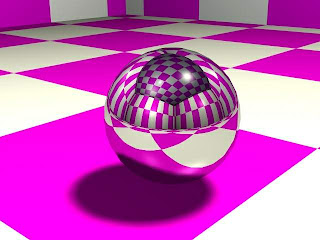Hence, is widely used in cartography (map building)http://construccion-de-mapas.blogspot.com/, And for example in celestial cartography:http://cartografia-celeste.blogspot.com/

Angles are also preserved in a transformation from outer space inside the sphere, that is, investment in the field of space: http:// tangencies-inversion.blogspot.com /

Non-Euclidean geometry of the sphere: http://geometria-de-la-esfera.blogspot.com/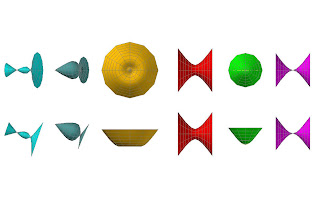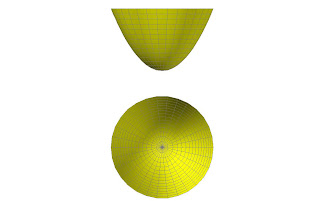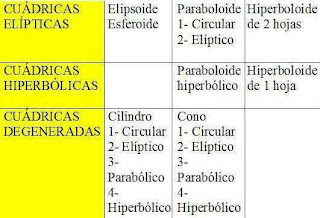http://geometria-poliedros.blogspot.com

page on regular polyhedra:http://nexmargul.260mb.com/
Click on the link to download a manual on regular polyhedra:http://www.box.net/shared/lpxg66qqvg ISBN.: 978-84-690-9786-1 Edit VA Com.Sa SC 2004 Examples of transformation of polyhedral surfaces:http://inscripcionpoliedrica.blogspot.com/

http://dodecaedro-regular.blogspot.com/
http://icosaedro-regular.blogspot.com/

Development of a surface.

The development of a surface is obtained on the plane figure spread on a plane. Is as if it were a figure surrounded by a fine material on its surface to be opened along the edges. As you open each of their faces the doblaríamos to place them in a plane of the drawing, being extended envelope of the figure on the plane. In developing the figure on a plane to obtain the true size and shape of faces. types of developments : A figure can be developed through parallel lines as with the improper vertex radiated surfaces, prisms and cylinders. may develop by lines passing through a center, also called radiation therapy. This development corresponds to point itself radiated surfaces such as cones and pyramids. By triangulation, this is obtained by dividing the surface sides of the triangular shapes. Developments are approximate to those used and warped surfaces of double curvature, surfaces can not be developed but can get rough forms of its length on the plane. Develop a surface is spread on a plane, is to take their faces and putting anexionarlas on a plane bound for his sides to turn it into a flat shape, so that if the edges are cut and folded over the sides can be constructed in three dimensions. polyhedral Figures are all developable. In the figure we see the development of a regular icosahedron, which you can see its 20 faces that are triangles equilateral.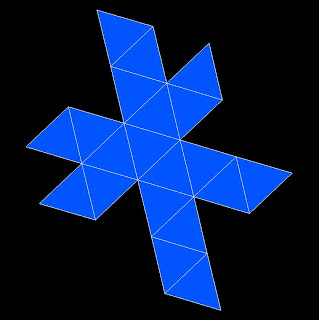Here we see the development of another surface, the cuboctahedron rombitruncado. This surface is an Archimedean polyhedron obtained by section of the faces of a regular polyhedron.Schlegel diagram. If we look at a figure very close to glass so that all edges are behind a face, we will see the figure depicted in this diagram. To make shapes according to this procedure has to be the case that the edges do not intersect each other and simultaneously display all vertices and edges of the figure in its representation. Provided that in this diagram polyhedral surfaces, the control vertices, the lines that pass through each vertex, side, etc..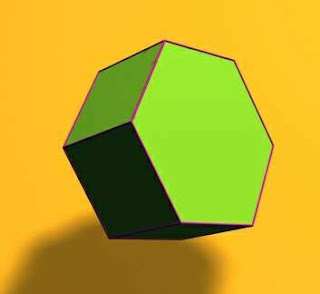Regular hexagonal prism base right. A prism is a polyhedron circumscribed developable surface. If the side edges connecting the bases are oblique with respect to them we are talking about an oblique prism, if not, speak of a right prism.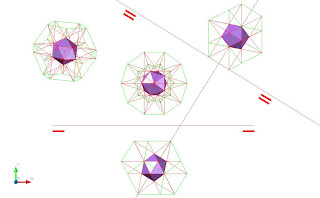Dual polyhedra as the icosahedron and dodecahedron have a curious property: if we extend the regular icosahedron edges intersect at vertices that determine the points of a dodecahedron. Conversely, if we extend the edges of the regular dodecahedron vertices are cut to determine the points of an icosahedron. Both figures, along with the hub where you can register, its edges are related in a golden ratio .Here we see an axonometric projection of the icosahedron to which they haveextended their edges and their intersection is generated by the vertices of a regular dodecahedron. By adding the pyramids we have obtained the great stellated dodecahedron . therefore can be obtained by incorporating a polyhedron star pyramids in each of the faces of a regular polyhedron, so on the icosahedron with pyramids on their faces also enroll vertices a regular dodecahedron.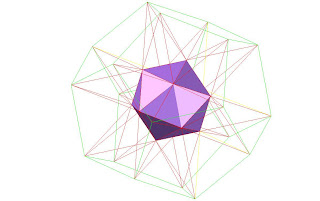The deltahedrapolyhedral developable ruled surfaces are formed by equilateral triangles. They may be regularly or irregularly. In this case the deltaedro is a regular icosahedron. The regular tetrahedron and the regular octahedron are two other regular polyhedra are also deltahedra.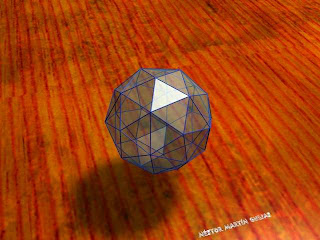Geodesic spheres are appropriate polyhedral surfaces areas. Can be constructed starting from a regular polyhedron in which are triangulated faces. Each new triangulation of the new vertex we get, must be registered in the area, generating more homogeneous surface possible, although this is sometimes not possible to produce surfaces which have not always triangles are all equilateral.http://estructuras-geodesicas. blogspot.com /

The figure shows how you can transform an Archimedean polyhedron(truncated icosahedron) in a geodesic sphere , that is, a structure built with nearly equilateral triangular faces, so that all vertices of the triangles of the sphere are equidistant from the center of the itself. On each of the faces of a pyramid is constructed figure whose base is the same face. To find the height must go through the midpoint of the face also a line passing through the center of the sphere that inscribes the polyhedron. This line will cut the sphere in which the polyhedron is inscribed in a point and this is the apex of the pyramid. Then join this vertex to each of the vertices of the base polygon vertices are regular (Archimedean polyhedron faces). Thus we have that all points of the geodesic sphere equidistant from the center and all its faces are equilateral triangles almost. If instead of taking as apex of the pyramid the intersection of the sphere with the line through the center of the sphere and the midpoint of each side we take a point farthest away over each face of the polyhedron, we get a star polyhedron . Thus we need to build a polyhedron crashed all we need is to build pyramids supported in each of the faces of the polyhedron.Archimedean polyhedraare developable ruled surfaces produced in most cases by sections of the regular polyhedra. Fairly regularly and have all sides of the Archimedean polyhedra are regular polygons.

On the next page we see the construction of rhombicosidodecahedron (p. Archimedean) from the truncation of an icosahedron: http://transformaciondesuperficies.blogspot.com/

Page on which you can see the construction of several Archimedean polyhedra from simpler ones, as the regulars. Transformation of a regular polyhedron (icosahedron) in different Archimedean polyhedra and back to another regular polyhedron: the dodecahedron.

http://poliedrosarquimedianos.blogspot.com/
http://arquimedianosendiedrico.blogspot.com/
http://poliedrosregularesyarquimedianos.blogspot.com/Here is an example of an Archimedean polyhedron generated by the truncation of the regular icosahedron .
It is the figure used in the classic soccer balls, it was thought until recently that no great number of edges had a sphericity, today others use a spherical structure.The Catalan polyhedraare duals of the Archimedean surfaces. Can be obtained by taking the midpoints of the Archimedean polyhedrons and joining them together.There is the peculiarity of these polyhedra are on all sides equal but irregular polygons (sides and angles).In the figure we see a Catalan polyhedron (hexecontaedro pentagonal icosidodecahedron dual blunt) in dihedral system.The star-polyhedra can be constructed in general by adding pyramids to the faces of polyhedra. The most studied are the small stellated dodecahedron, great dodecahedron, the great stellated dodecahedron and the great icosahedron. Can be transformed into each other: the great dodecahedron in small stellated dodecahedron and great dodecahedron each other and crashed into the great icosahedron and vice versa.Were studied by Kepler, Poinsont, Cauchy, and so on.http://poliedroestrellado.blogspot.com/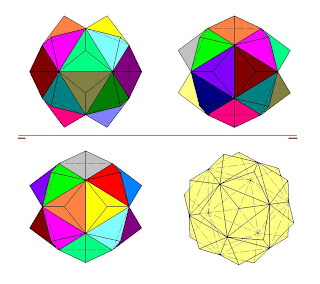In the figure we see in dihedral system and axonometric view a regular icosahedron which have been added pyramids on their faces, thereby obtaining a polyhedron crashed.
If the addition of the pyramids is such that the sides match the extension of the three faces that surround each of the faces of the icosahedron, we get thefirst star of the icosahedron . Same with the dodecedro and its corresponding first star of the dodecahedron. At cut 2 dual polyhedra by cutting type 1, you get always the same polyhedron . In the case of the icosahedron, as dual dodecahedron, when cut by planes passing through the center of the ridge (is type 1, 2 would be for a third of the edge) have the icosidodecahedron in pink and defined lines in the picture (just as we get to cut the dodecahedron). If each of the pentagonal faces of the icosidodecahedron, join the vertices of the pentagons together, we have figures called staves, in the drawing in yellow. If each side of the staff do planes parallel to each set of three staves correlated, removing the prism determining these three planes we obtain the figure drawn in dihedral system, which is a great dodecahedron truncated by its vertices.Al prolong the faces of the first star of the great dodecahedron dodecahedron is obtained. If in this figure achaflanamos vertices, we obtain the previous polyhedron is now appearing at the end of the video, that is, the large truncated dodecahedron . Figure observe the transformation of a small dodecahedron crashed into a large dodecahedron . First achaflanamos the vertices of the dodecahedron pentagonal star pyramid pentagons and then getting staves arise each pentagon of the figure, staves determined by the prisms are actually an extension of the faces of the dodecahedron. As these staves are transformed into a point, we have been extended to the boundary faces of the first stellated dodecahedron, thereby obtaining faces are regular pentagons on which sits a volumetric star, whose arms are used for the adjacent star . If we take a prism and one of its bases to match the turn to a vertex in a mid-point orthogonal to the edge of the other base, using an orthogonal projection, we get an antiprism . The rotation must be such that looking at both sides antiprism in parallel the plant, both show its vertices always equidistant from each other. The antiprism is formed by joining two vertices of regular polygon whose center of both bases affects an orthogonal axis, by zig-zag lines which determine the triangles same side of the figure. A antiprism in an oblique position in the dihedral system.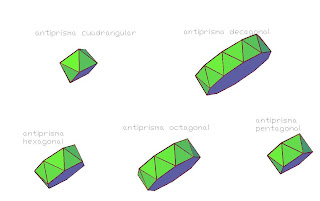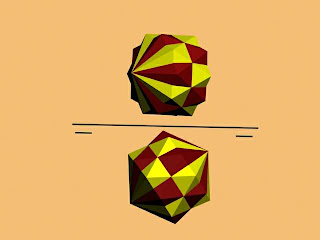Polyhedra compoundsare those which are formed by several polyhedrons which have the same center.The picture shows two regular icosahedrons obtained by a rotation relative to each other on one axis passing through the vertices.http://poliedroscompuestos.blogspot.com/

Adding pyramids to the faces of an octahedron we get a polyhedron composed of tetrahedra.Johnson polyhedra are those that remain convex and without regular or Archimedean, are formed by faces that are regular polygons.Johnson built all the polygons that fall within this classification. Pictured are a few.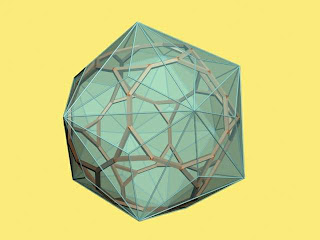The truncated dodecahedronTriaquisicosaedro two dual polyhedra , the Archimedean can register in the form of Catalan in the latter we take the midpoints of the sides and join them.http://icosaedrotriakis.blogspot.com/

Obtaining icosaedrotriakis by incorporation of pyramids to the faces of the regular icosahedron.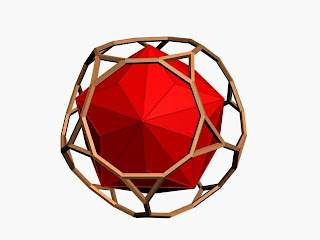We note that the converse is not true and triaquisicosaedro may not register in the truncated dodecahedron so that the vertices of the polyhedron of Catalan are on the midpoints of the faces of Archimedean.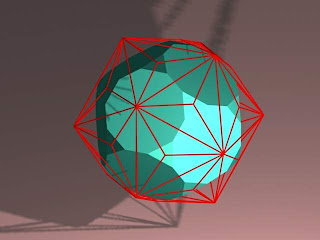Here is another view in which the Archimedean is enrolled in the Catalan. Thetriaquisicosaedro is nothing that the icosahedron with pyramids on their faces, hence its name with the prefix Triakis-name designating Catalan polyhedra in this detail.vertices on edges: A regular octahedron can be inscribed in a regular tetrahedron so that the vertices of the first bearing on the midpoints of the edges of the other. This is another way to construct new polyhedra from the midpoints of each edge.If we take the midpoints of the faces of an antiprism and unite with their closest vertices, we obtain a dual figure which is the deltoedro antiprism . It is a figure used in dice, and you can have unlimited number of faces to be homogeneous and all sides be equally available to the center, there are as likely to drop any face in a roll of the dice.Axonometric projection of adeltoedro , consists of delta shaped faces, trapezoids with an axis of symmetry.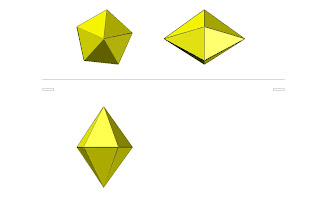Here we see a dipyramid is a polyhedron formed by two pyramids joined together, dual prism obtained at the junction of the central vertex of each face of the prism.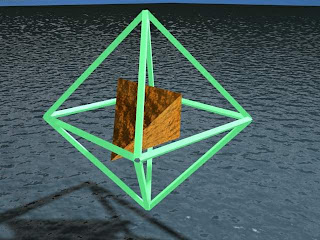Vertices on faces:Another possible registration of regular polyhedra: a regular tetrahedron inscribed in a regular octahedron, the latter taking the midpoints of some faces (of all we would have in this case the cube, its dual). As the two polyhedra are dual not we take all points of each side but a few.

Some theorems of geometry that are frequently used in the dihedral system: